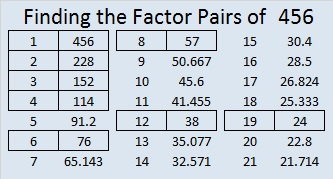# 456 An Inchworm Measuring Marigolds

456 is the sum of consecutive prime numbers in two different ways. One of my readers listed those ways in the comments. The factors of 456 are at the end of the post.Inchworm, inchworm,
Measuring the marigolds
You and your arithmetic will probably go far.

Two plus two is four
Four plus four is eight
Eight and eight is sixteen
Sixteen and sixteen is thirty-two.

Inchworm, inchworm,
Measuring the marigolds
Seems to me you’d stop and see
How beautiful they are.

Today I taught a class of three year olds about being thankful for birds, insects, and creeping things. To keep their attention, I used a variety of stories, riddles, books, and games. I also sang a few songs including this one about an inchworm who is very good at arithmetic. I think preschool children can still enjoy songs like this even if they don’t understand everything the song is about or even if they are wiggling as much as an inchworm while they listen to it. Here is the song sung by Danny Kaye from the movie Hans Christian Andersen:

———————————————————————————————————

Now for the number 456. The last two digits can be evenly divided by four, so the entire number is divisible by four. Also since it is formed from three consecutive numbers, it is divisible by 3. However since the number in the middle of those consecutive numbers is not 3, 6, 9 or another multiple of 3, we know that 456 is NOT divisible by 9.

Because it is divisible by four, we will use that fact first to determine how to reduce its square root.456 ÷ 4 = 114. Notice that 114 is even, but 14 can’t be evenly divided by 4, so 114 cannot be either. Also notice that 114 is still divisible by 3. If we’re not sure whether or not 114 has any square factors, we are less likely to make a mistake if we divide it by 6 once, instead of by 2 and then by 3.114 ÷ 6 = 19, a prime number, and we are certain there were no other square factors. Since we know 19 x 6 = 114, let’s backtrack a little and go back to that original one layer cake:Take the square root of everything on the outside of the cake and get √456 = (√4)(√114) = 2√114

———————————————————————————————————

• 456 is a composite number.
• Prime factorization: 456 = 2 x 2 x 2 x 3 x 19, which can be written 456 = (2^3) x 3 x 19
• The exponents in the prime factorization are 3, 1, and 1. Adding one to each and multiplying we get (3 + 1)(1 + 1)(1 + 1) = 4 x 2 x 2 = 16. Therefore 456 has exactly 16 factors.
• Factors of 456: 1, 2, 3, 4, 6, 8, 12, 19, 24, 38, 57, 76, 114, 152, 228, 456
• Factor pairs: 456 = 1 x 456, 2 x 228, 3 x 152, 4 x 114, 6 x 76, 8 x 57, 12 x 38, or 19 x 24
• Taking the factor pair with the largest square number factor, we get √456 = (√4)(√114) = 2√114 ≈ 21.3542———————————————————————————————————

Picture credits: Inchworm and ruler: http://www.kindergartenkindergarten.com/2012/06/problem-solving-measurement.html;